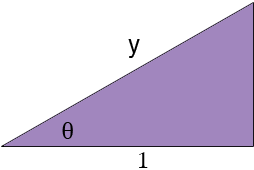SEARCH HOMEMath Central Quandaries & QueriesQuestion from Tom, a student: I am having trouble with this question. For y > 1, the value of cosec [arccos (1/y)] is? So far i have let arccos (1/y) = theta so , cos theta = 1/y The answer is y/ sqroot (y*2 - 1), what are the steps in getting this answer, and is there a general rule for working these out? Thankyou.Hi Tom,

I would draw a diagram. If arccos (1/y) = theta thenUse Pythagoras theorem to find the length of the third side of the triangle. From the diagram what is cosec(θ)?

PennyMath Central is supported by the University of Regina and The Pacific Institute for the Mathematical Sciences.